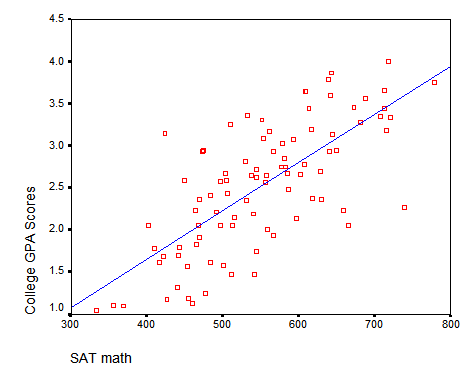# Tensorflow 2 for Deep Learning -Linear Regression

Code files will be available at : https://github.com/ashwinhprasad/Tensorflow-2.0

# What is Linear Regression ?

Linear regression is basically using a equation of a line to find out the linear relationship between 2 variables. By finding the linear relationship, I mean finding the parameters ( Slope and Intercept).

y = m*x + c
y : dependent variable
x : independent variable
m : slope
c : interceptexample of linear relationship between 2 variables

In the above image , SAT math is the Independent variable and College GPA scores is the dependent variable and it is clear that there is a linear relationship between these 2 variables. hence, we can use a line and predict college gpa scores for new students given thier sat math , once we found slope and intercept.

# Linear Regression with Tensorflow 2

## 1. Importing the required Libraries

`#importing the librariesimport tensorflow as tfimport pandas as pdimport numpy as npimport matplotlib.pyplot as pltimport seaborn as sns`

## 2. Importing the Dataset

Importing the dataset. This dataset is a simple dataset from sklearn. you can use any dataset where the independent variable and dependent variable have a linear relationship

`#data preprocessing#train setdata_train = pd.read_csv('train.csv')data_test = pd.read_csv('test.csv')#removing null valuesdata_train = data_train.dropna(axis=0,how='any')`

## 3. Train Test Split

Splitting the data into training and test set

`#xtrain, x_test , y_train, y_testx_train = data_train['x']y_train = data_train['y']x_test = data_test['x']y_test = data_test['y']`

## 4. Plotting the data

`plt.figure(figsize=(8,6)) plt.scatter(x_train,y_train)`

## 5. Converting the data to numpy

`#converting to numpyx_train = np.array(x_train).reshape(-1,1)y_train = np.array(y_train)print(x_train.shape,y_train.shape)output: (699, 1) (699,)`

## 6. Creating a Sequential Model with TF2

Sequential Layer allows stacking of one layer on top of the other , enabling the data to flow through them

There are 2 ways to do this as shown in the below cell

`from tensorflow.keras.models import Sequentialfrom tensorflow.keras.layers import Densemodel = Sequential()model.add(Dense(1))"""(Alternative way for dedfining a sequential model)model = Sequential([        Dense(1)])"""`

## 7. Optimizer and Gradient Descent

we use mini-batch gradient descent optimizer here. and mean square loss for this problem

`from tensorflow.keras.optimizers import SGDfrom tensorflow.keras.losses import msemodel.compile(optimizer=SGD(learning_rate=0.0001),loss=mse)train = model.fit(x_train,y_train,epochs=50)`

## 8. Performance Analysis

The loss is reduced over time and the line of fit of the model seems to be the best fit for the below data

`#loss over timeplt.plot(train.history['loss'],label='loss')plt.xlabel('epochs')plt.ylabel('loss')plt.legend()`

## 9. Testing the Model on the Test Set

`#testing the modely_pred = model.predict(np.array(x_test).reshape(-1,1))plt.figure(figsize=(10,8))plt.plot(x_test,y_pred,color='red',linewidth=2,label='Line of best Fit')plt.scatter(x_test,y_test,label='Test data plots')plt.legend()`

# Conclusion

The model learns these parameters (slope and bias) from an algorithm called gradient descent. To learn how gradient descent works , check out : https://medium.com/analytics-vidhya/linear-regression-with-gradient-descent-derivation-c10685ddf0f4.
So, The parameters of the line of best fit would be in such a way that the difference between the points in the line and the sum of data points that are vertically away from it is minimum. This can be better understood by going through the gradient descent blog post.

Once we get the equation of this new line, we can predict y’s for new value of x’s .

--

--